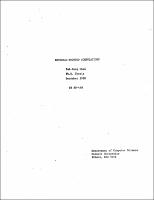## Reversal-Bounded Computations##### Abstract
IN computations by abstract computing devices such as the Turing machine, head reversals are required for searching the input or retrieving intermediate results. Hence the number of head reversals is a measure of the complexity of a computation. This thesis is a study of reversal-bounded computation on three models of abstract computing devices. The first model is the 1-tape Turing machine with finite bounds on head reversals. It is known that such machines recognize exactly the regular sets so that for recognition purposes, reversals can be eliminated entirely. For transduction purposes, that is, if an output is expected on the tape, a single reversal suffices. Hence these machines are most appropriately called finite automata. Clearly they are among the weakest possible computing devices, and many decision problems about them are solvable. We use this fact and a very simple input-output encoding scheme to obtain greatly simplified proofs of the decidability of some weak mathematical theories, including the weak monadic second-order theory of one successor and Presburger arithmetic. Similar techniques yield linear size bounds as well as linear time complexity bounds (on the multitape Turing machine model) for functions definable in Presburger arithmetic. As corollaries, we find applications in linear diophantine systems and linear integer programming. The second model is the multicounter machine with general bounds on counter reversals. By relating counter reversal to time and space, we show that recursiveness of reversal bounds implies recursiveness of the sets accepted. For bounds that are at least linear, counter reversal is polynomially equivalent to Turing machine time in both the deterministic and the nondeterministic cases. This result leads to a general deterministic reversal hierarchy and a natural formulation fo the P=?NP question on the multicounter machine model. It also suggests that on every reasonable universal computing model, there is a "natural" complexity measure that is polynomially equivalent to Turing machine time. For reversal bounds that grow more slowly than $n^{1/2}$, we show that the nondeterministic 2-way reversal complexity class is not closed under complementation and strictly includes the corresponding deterministic class. In contrast, the analogous questions are open for 2-way 2-tape Turing machines with logarithmic space bounds. Finally, we consider finite bounds on counter reversals. For both the 1-way and the 2-way models, we obtain very sharp upper bounds on the Turing machine time and space complexity of the languages accepted. In the 1-way case, we present a unified account of the known results, interspersed with our contributions, which include (1) equivalence to 1-way simple multihead finite automata, (2) an easy technique for proving nonrecognizability, (3) an abstract characterization of the power of finite reversal-bounded counters, and (4) an application to the theory of program schemes. Lastly, we consider finite reversal-bounded multitape finite automata. We show that over a single-letter alphabet, the languages accepted are exactly the unary encodings of Presburger relations. This result holds whether the model is determinisitic or nondeterministic, and even if it is augmented with, for example, finite reversal-bounded counters and an unrestricted pushdown store, or if the reversals are restricted to rewinds, that is, instructions that simultaneously position all heads at the beginnings of their respective tapes. For both deterministic and nondeterministic rewind automata, we establish exhaustive hierarchies based on the finite number of rewinds. When restricted to a single-letter alphabet, the deterministic hierarchy stands but the nondeterministic one collapses.
1980-12
##### Publisher
Cornell University
##### Keywords
computer science; technical report
##### Previously Published As
http://techreports.library.cornell.edu:8081/Dienst/UI/1.0/Display/cul.cs/TR80-448
technical report